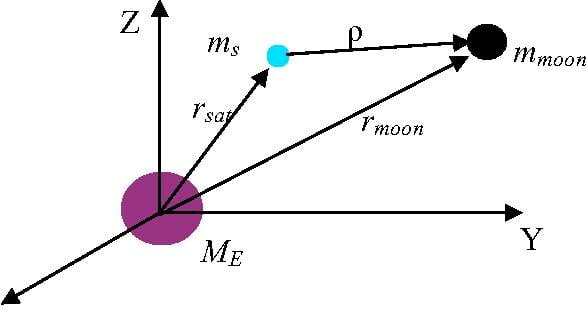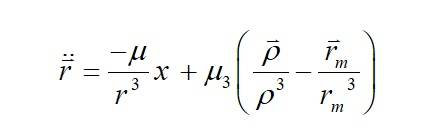# Change the value of a variable at each time step in matlab?

• MATLAB
• patric44

#### patric44

TL;DR Summary
is it possible to Change value of variable at each time step?
hi guys
I am trying to implement the a second order differential equation tat contains a time dependent term, the equation looks something like
r'' = -\mu/r^3+(g/g^3-m/m^3)
the idea is that i want to calculate 'r' the position vector of a point, that is dependent on vectors g and m, I tired to crate an simple ode integrator to get the g vector as

Matlab:
function dx = themoonpos(t,x)
R = 6378.137;
u = 398600.441800; % km/s2
% J2 = 0.0010826269;
% b = -1.5*J2*u*R^2 ;
r = sqrt(x(1)^2+x(3)^2+x(5)^2);
%% the propagator
dx=[
x(2)
;-u*x(1)/(r)^3
;x(4)
;-u*x(3)/(r)^3
;x(6);
-u*x(5)/(r)^3];
end

than i tried to implement that in another function dx that will be integrated as

Matlab:
function dx = thethirdp(t,x,yk)
k0 = [
-120901.611171555;
89921.008706315/(24*60*60);
-298392.399263114;
-25049.485694581/(24*60*60);
-162652.182605121;
-12799.914191783/(24*60*60)
];

opts = odeset; opts.RelTol = 10^-9;
[t2,yk] = ode45(@themoonpos,[0:60:5000],k0,opts);

R = 6378.137;
u = 398600.432896939; % km/s2
um = 4902.80058214776; % km/s2
J2 = 0.0010826269;
b = -1.5*J2*u*R^2 ;
r = sqrt(x(1)^2+x(3)^2+x(5)^2);
xm = (yk(1)-x(1));
ym = (yk(3)-x(3));
zm = (yk(5)-x(5));
rd = sqrt(yk(1)^2+yk(3)^2+yk(5)^2);
rm = sqrt(xm^2+ym^2+zm^2);
%% the perturbation
apx = um*((xm/((rm))^3)-yk(1)/(rd)^3);
apy = um*((ym/((rm))^3)-yk(3)/(rd)^3);
apz = um*((zm/((rm))^3)-yk(5)/(rd)^3);

%% the Integrator
dx=[
x(2)
;-u*x(1)/(r)^3+apx
;x(4)
;-u*x(3)/(r)^3+apy
;x(6);
-u*x(5)/(r)^3+apz];
end
then this function is integrated using the ode45.
the thing is the values yk i don't know how to assign it to each time step that the integrator uses, is this the right thing to do it
I hope anybody help i tried to post this on mathwork but no answer

Maybe I am misunderstanding your question, but can't you just use the "usual" trick of introducing a dummy variable equal to r' (e.g. set x=r')and then solve the resulting system of two equations?
This should be well described in the manual

Generally speaking, when solving a 2nd order ODE you always end up solving a system of two equations (for 3rd order you would get 3 etc)

Maybe I am misunderstanding your question, but can't you just use the "usual" trick of introducing a dummy variable equal to r' (e.g. set x=r')and then solve the resulting system of two equations?
This should be well described in the manual

Generally speaking, when solving a 2nd order ODE you always end up solving a system of two equations (for 3rd order you would get 3 etc)
the problem is that my 2nd order ode has a time dependent term that comes from another solution of a 2nd order ode, so I am trying to solve first for the first one and get a column vector of solutions, then plug that back into my other integrator to get the original "r" I want. but it doesn't seem to work
what is the usual way to do that?

Sorry, I still don't get it
Are you saying you have two coupled 2nd order ODEs?
I initially assumed that you should be able to rewrite this as a system of 1st order ODEs
I.e. similar to what is done in the example of the van der Pol equation in the Matlab documentation

https://uk.mathworks.com/help/matlab/ref/ode45.html

Are you saying you can't re-write your problem as a system of 1st order ODEs for some reason?

•patric44
the problem is that my 2nd order ode has a time dependent term that comes from another solution of a 2nd order ode, so I am trying to solve first for the first one and get a column vector of solutions, then plug that back into my other integrator to get the original "r" I want. but it doesn't seem to work
what is the usual way to do that?

Isn't that just the single system
\begin{align*} \ddot g &= F_g(g) \\ \ddot x &= F_x(x,g) \end{align*}

•patric44i am trying to solve this equation which take the third body perturbation into accountseparating each compoent I am left with 3 second order equations for each component with rm as a time dependent variable also, what is the right way to solve this ?

If you have 3 2nd order ODEs you should probably re-write this as a system of 6 1st order ODEs
When you say that rm is a time dependent variable; do you mean that it is an independent variable (no dependence on r) which changes over time?

•patric44
If you have 3 2nd order ODEs you should probably re-write this as a system of 6 1st order ODEs
When you say that rm is a time dependent variable; do you mean that it is an independent variable (no dependence on r) which changes over time?
i meant that the position of the moon is dependent on time, but I guess I can solve them as you guys said as a whole 6 second order coupled equations. I will try that hope it worksIf you work in the inertial frame in which the centre of mass is fixed at the origin, then you can reduce the number of equations by a third since one of the positions can be determined from $$0 = m_1\mathbf{r}_1 + m_2\mathbb{r}_2 + m_3\mathbf{r}_3.$$

•patric44